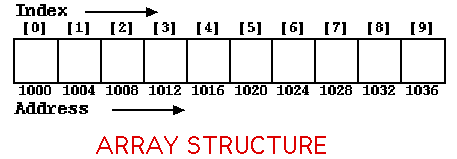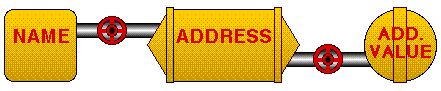# NOTE: This set of www pages is not the set of www pages for the curent version of COMP101. The pages are from a previous version that, at the request of students, I have kept on line.

## CONTENTS

 1. Introduction to compound data types 2. Arrays 2.1. Features of arrays 3. Array declaration 4. Assignment 5. Example problem - metres to yards, feet and inches conversion ` ` 5.1. Requirements 5.2. Analysis 5.3. Design 5.4. Implementation 5.5. Testing

Note: The programming example given here makes use of a default constructor.

## 1. INTRODUCTION TO COMPOUND DATA TYPES

 The available primitive (basic) types can be extended by adding compound types made up of existing primitive types (and/or other compound types). Compound types are usually programmer defined. ` ` There are some standard compound types which available in many (object oriented and imperative) programming languages. These include: Arrays Strings We will be discussing both of these.

## 2. ARRAYS

The most straight forward (and oldest) form of compound data type is the array. All the types we have considered so far have been scaler (data items of the types considered can only have one value). In an array a data item consists of a numbered collection of similar items. As such it can be viewed as simply a collection of data items, all of the same type, stored in a series of locations in memory such that a single value is held at each location This is illustarted in Figure 1 where we have an array intended to store a collection of 10 data items.Figure 1:

### 2.1. Features of arrays

 Items in arrays are called elements (some authors use the term cell to describe the locations within an array). The number of elements in an array is described by its length. The array in Figure 1 has a length of 10. Specific elements in an array can be identified through the use of an index. ` ` In Java the index is always of the type integer (this is not the case in all programming languages). In Java the first index is always the integer 0 which is referred to as the lower bound, the last index is then referred to as the upper bound. The upper bound for the array given in Figure 1 is 9. Note that: upperBound = length-1

## 3. ARRAY DECLARATIONS

 When declaring arrays we are doing two things: Declaring the type of the array, i.e. the type of the elements to be contained within it. Declaring the array length (and by extension the upper bound, which is the array length minus one --- indexing starts at 0). In Java, to declare an array data item we use a declaration statement of the form: ```< TYPE_NAME > [] arrayName; ``` Without the [] this tell the Java compiler to create space for an integer data item called arrayName. The [] then indicates that this is actually the start of an unspecified array of integers. The value associated with the array name is an address, i.e. a reference value (Figure 2), that marks the start of a block of memory. So that an appropriate amount of memory can be set aside we must also tell the compiler how many elements to expect. We do this as follows: ```< TYPE_NAME > [] arrayName = new < TYPE_NAME > [N] ``` where N is the number of elements. Note the similarity between the above and the way we declared an object: ```< CLASS_NAME > objectName = new < CONSTRUCTOR > ( < ARGUMENT_LIST > ) ``` ` ` In many ways we can also consider a class to be a compound type. Let us consider an example of an array declaration: ```int [] temperatureArray = new int; ``` Here we have created an array of 10 integers called temperatureArray (presumably to store a sequence of temperatures in). Alternatively, if we wish to store our temperatures as doubles we would have declared the array as follows: ```double [] temperatureArray = new double; ``` Note that if we has not used an array to store our temperatures we would have had to declare 10 distinct data items: ```double temperature0; double temperature1; double temperature2; double temperature3; double temperature4; double temperature5; double temperature6; double temperature7; double temperature8; double temperature9; ``` Very tedious for small numbers of elements and completely impractical for any number of elements beyond (say) 50.Figure 2: Object reference data item

## 4. ASSIGNMENT AND INITIALISATION

 Assignment: Given knowledge of the index for an array element we can assign a value to that element (or change its value) using an "assignment" operation. Example: ```myArray = 4; ``` Here we have an array data item (named myArray) and we have assigned a value of 4 to the first element of this array (i.e. the element at index 0). Note how we have specified the element we are interested in by indexing into the array. ` ` Initialisation: Remember that initialisation is the process of assigning an "initial" value to a data item on declaration. Java (along with many other programming languages) supports the concept of compound values (also sometimes referred to as array aggregates) which allow all the elements of an array to be assigned to simultaneously on initialisation: ```int[] myArray = {9,8,7,6,5,4,3,2,1,0}; ``` Note that we do not need to define the length in this case as this can be deduced by the Java compiler.

Created and maintained by Frans Coenen. Last updated 10 February 2015